# X-Ray Spectra and the Distribution of Energetic Electrons

Spectra are crucial for determining the physical conditions of the particles producing the observed radiation. Are the particles thermal or nonthermal? If they are thermal, what is their temperature and density? If they are nonthermal, how many have been accelerated, to what energies, and how are they distributed in energy? The answers to these and related questions are necessary to determine how the flare energy is released and where it goes.

The top figure in the table below shows x-ray spectra from the cusp and from the left and right footpoints of our model loop when the plasma density in the loop is 2 x 1011 cm-3. The bottom figure shows how the energetic electrons that are radiating the nonthermal x-ray emission are distributed in energy. The spectra were obtained by calculating the flux of radiation at many photon energies from a single 1 arcsec by 1 arcsec area near the top of the cusp and within each footpoint.

The spectra and electron distributions are shown on log-log plots. This means that each marked interval on both axes of the graphs is a factor of 10 greater than the previous interval. This allows us to show a much greater range of photon fluxes (or energetic electron densities) and photon (or electron) energies on a single plot than would be possible with a linear scale. It also has the advantage that a power law (see The Injected Electrons) appears as a straight line on the plot.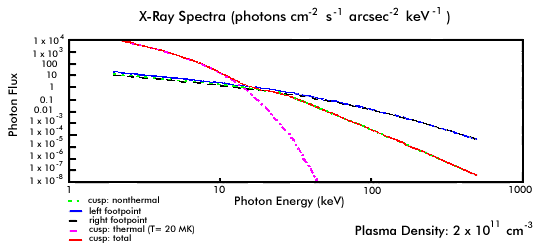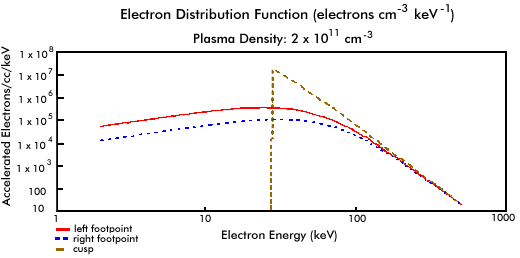The electron distribution at the location in the cusp (dashed brown curve) is nearly identical to the injected electron distribution. Some energy loss by the lowest energy electrons is barely visible (the curve falls slightly below the straight-line power law of the injected electrons in the 30 keV to about 100 keV range). The corresponding x-ray spectrum (dashed green curve) is a double power law: a power law above 30 keV and a flatter power law below 30 keV. The slope of the spectrum above 30 keV is determined by how rapidly the electron distribution falls off with increasing energy: an electron distribution for which the number of electrons falls off rapidly with increasing energy (a steep or "soft" distribution) produces a steeper x-ray spectrum than a distribution that does not fall off rapidly ( a flat or "hard" distribution). The x-ray spectrum is flatter below 30 keV because these x-rays are only produced by electrons with energies above 30 keV. The slope of the spectrum below 30 keV is determined by the bremsstrahlung process.

Below around 10 keV the x-ray spectrum from the cusp is dominated by the thermal radiation from the 20 million degree plasma (violet curve). The total spectrum from both the thermal and nonthermal components (red curve) is similar enough to a single or double power law that it would be difficult for a detector with low spectral resolution to distinguish the two components.

The effects of energy losses are apparent in the electron distributions from the two footpoints. The greater distance to the right footpoint shows up in the electron distribution (dashed blue curve) as greater energy losses when compared to the electron distribution from the left footpoint (red curve). Since the losses are greatest for lower energy electrons, the difference between the two distributions is less at higher energies. This is reflected in the x-ray spectra from the left (blue curve) and right (dashed black curve) footpoints.

The relatively flat x-ray spectra from the footpoints above 30 keV are not what is expected from the footpoint electron distributions shown in the lower figure. The lower figure shows how the local density of energetic electrons is distributed in energy. It shows the electron distribution in only one cubic centimeter of the loop volume. In the footpoints, however, the electron energy distribution is changing very rapidly with position along the loop because the collisional energy losses are very large. The x-ray spectrum is from all the energetic electrons along the line of sight that are contained within an area that is 1 arcsec square (725 km by 725 km). Therefore, the x-ray spectrum is determined by the sum of all the electron distributions within the 1 square arcsec resolution of the observation. This includes electrons that have suffered only minor energy losses as well as electrons that have suffered extensive energy losses. The result is the flatter x-ray spectrum shown in the upper figure. This type of spectrum is often called a thick-target spectrum, because the x-rays are emitted by electrons that lose all of their energy in the observed "target". The x-ray spectrum from the cusp, where energy losses are minimal and the electron distribution does not change significantly over the observed area, is often called thin-target.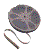View a video animation showing how the cusp and footpoint x-ray spectra change as the plasma density in the loop increases from 1 x 109 cm-3 to 5 x 1012 cm-3. The video is available in AVI (940 K) and MPEG (425 K) formats.View a video animation showing how the distribution of nonthermal electrons in the cusp and in the footpoints changes as the plasma density in the loop increases from 1 x 109 cm-3 to 5 x 1012 cm-3. The video is available in AVI (1000 K) and MPEG (380 K) formats.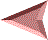Back: Variations with Plasma Density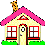Solar Flare Homepage